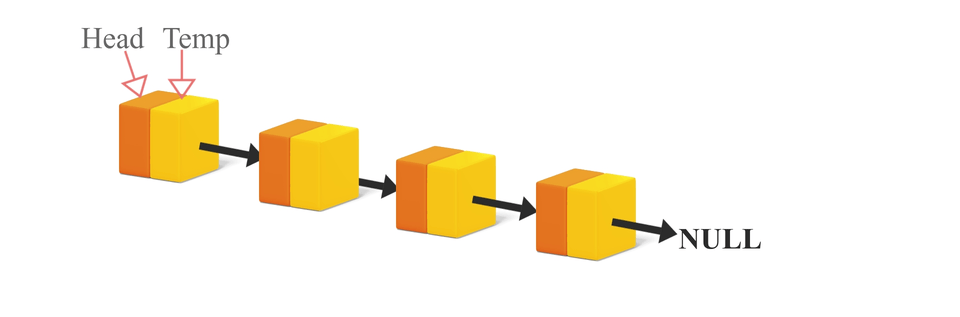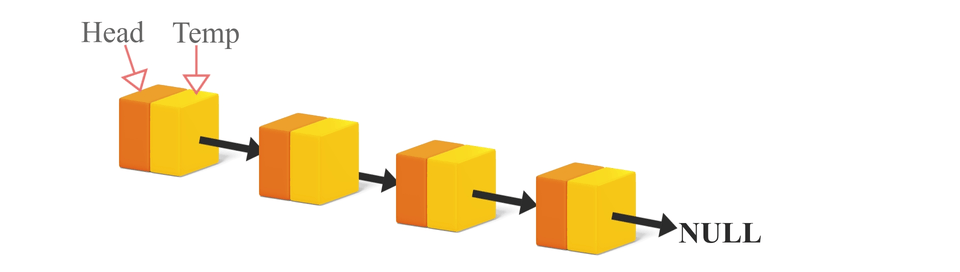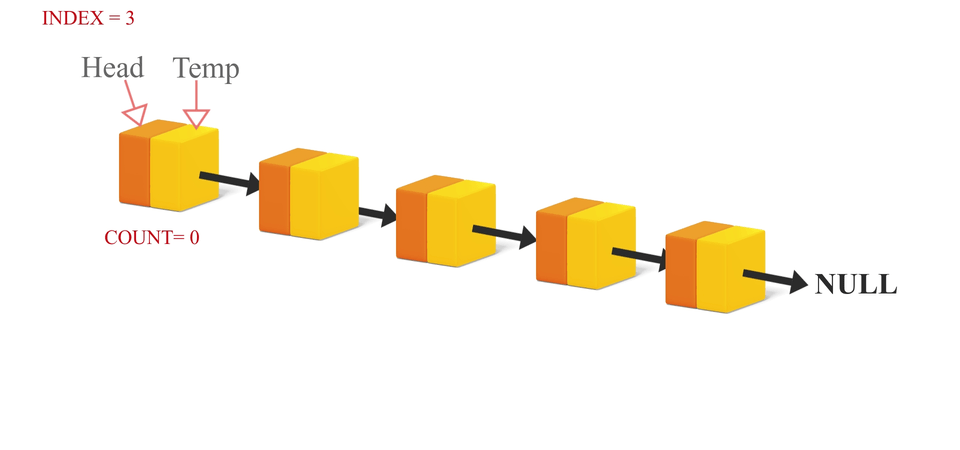Created June 16, 2021

In this blog I'm gonna be talking about the deletion operations in linked lists. Just like how there are three insertion operations in a linked list, there are three deletion operations. All the three deletion methods are just the reverse of what we do in insertion operations.

## The three types of Node Deletion

### 1. Delete node from the front

Here, we first set a temp variable equal to the head node. Then we shift head node to its next node and then we detach the first node from the list and deallocate the memory allocated to it. Finally, return the head node. The steps to follow are like this:

-> set temp equal to the head node.

-> shift head to the next node in the list.

-> set next of temp equal to null.

-> free the space allocated to temp.

The C code for the same is as follows:

``````NODE *deleteFromFront(NODE *head){
temp->next = NULL;
free(temp);
}
``````

An illustration of the process:### 2. Delete node from the back

In this type of deletion, we'll first traverse all the way to the second last element of the list and then set the next of this node to null.

The steps to follow are like this:

->traverse to the second last node of the list.

->set the next of second last node to null.

The C code looks like this:

``````NODE *deleteFromBack(NODE *head){
while(temp->next->next != NULL){
temp = temp->next;
}//temp reaches the second last node;
temp->next = NULL;
}
``````

If you want to free the memory allocated to the deleted node, you'll have to make use of a second pointer variable. Then the C code will look like this:

``````NODE *deleteFromBack(NODE *head){
temp1 = temp1->next;
while(temp->next->next != NULL){
temp = temp->next;
temp1 = temp1->next;
}//temp reaches the second last node;
temp->next = NULL;
free(temp1);
}
``````

An illustration for the same looks like this:### 3. Delete node at a specific index

This is a little bit trickier than the other two categories, but no worries! Once you get an idea, this will be a piece of cake for you!

So here it goes-

Firstly, we traverse to the node before the target node using counter variable. Then set the next of this node(node before the targeted node) to the next of the targeted node. Then we free the targeted node, now that it is detached from the linked list. The steps for the same are:

-> traverse to the node just before the target node. Lets call this node n1, the targeted node n0 and the next of the target node n2.

-> set the next of n1 to n2 and and the next of n0 to null.

-> now free n0.

It's code in C looks like this:

``````NODE *deleteAtIndex(NODE *head, int index){
int count=0;
while(count!=index-1){
temp = temp->next;
count++;
}//temp reaches the element before the target element
NODE *temp1 = temp->next->next;//next node of the target node
NODE *temp2 = temp->next;//the target node
temp2->next = NULL;
temp->next = temp1;//the next of node before the target node is set to the node next to the target node
free(temp2);
}
``````

Illustration of the process:## Conclusion

Deletion in Linked lists looks kind of challenging at first, but once you pick up the concepts, you will be able to do it without any difficulty. Both insertion and deletion operations require a clear visual idea of what exactly we're doing to the list and nothing else. I hope my explanation provided you that clear idea!

Happy learning!